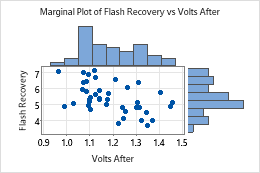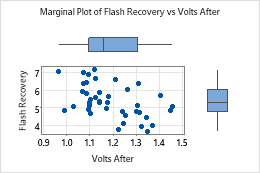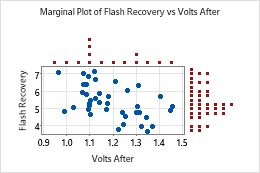# Select a marginal plot

Choose Graph > Marginal Plot, then select a marginal plot based on how you want the plot to look.

## With Histograms

Create a scatterplot with histograms of the x and y variables in the margins. For example, the following marginal plot shows the relationship between volts remaining in a camera battery after a flash and the flash recovery time, with histograms of the two variables in the margins of the graph.## With Boxplots

Create a scatterplot with boxplots of the x and y variables in the margins. For example, the following marginal plot shows the relationship between volts remaining in a camera battery after a flash and the flash recovery time, with boxplots of the two variables in the margins of the graph.